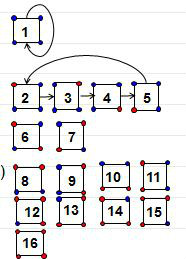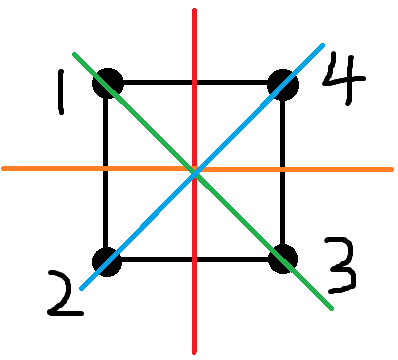# 漫谈OI中的群论入门

## 定义

S$S$是一个集合,$·$是一个二元运算符,并不是指乘号,比如平常我们在数集上所使用的+  × ÷$+\ -\ ×\ ÷$,集合运算中使用的 $∪\ ∩$都是二元运算

### 1.封闭性

x,yS,xyS$\forall x,y∈S,x·y∈S$

### 2.结合律

x,y,zS,(xy)z=x(yz)$\forall x,y,z∈S,(x·y)·z=x·(y·z)$

### 3.单位元

eS,xS,ex=xe=x$\exists e∈S,\forall x∈S,e·x=x·e=x$

S=Z,=+$S=Z,·=+$时,e=0$e=0$
S=R,=×$S=R,·=×$时,e=1$e=1$
S=N+,=$S=N_+,·=-$时,没有单位元

### 4.逆元

xS,yS,xy=yx=e$\forall x∈S,\exists y∈S,x·y=y·x=e$

S=Z,=+$S=Z,·=+$时,x1=x$x^{-1}=-x$
S=R,=×$S=R,·=×$时,x1=1x$x^{-1}=\frac 1 x$,所以这个二元组不是群,因为0不是逆元

xG$\forall x∈G$,aG,ax=e$\exists a∈G,a·x=e$,即a$a$x$x$的左逆元

bG,ba=e$\exists b∈G,b·a=e$

xa=(ba)(xa)=b(ax)a=ba=e$x·a=(b·a)·(x·a)=b·(a·x)·a=b·a=e$
a$a$也是x$x$的右逆元

## 相关概念

### 阶

G$G$中所含元素的个数,称为群G$G$的阶,记为|G|$|G|$,即S中所含元素的个数

(ak$a^k$表示连续k个a进行G$G$中规定的运算，不是传统意义上的k个a相乘)

### 消去律

b=ca+b=a+c$b=c\Leftrightarrow a+b=a+c$
b=ca×b=a×c  (a0)$b=c\Leftrightarrow a×b=a×c\ \ (a≠0)$

t就是a的逆元了

### 置换群

n个元素1,2,…,n之间的一个置换为
(1,2,3,...,na1,a2,a3,...,an)$\begin{pmatrix} 1,2,3,...,n \\ a_1,a_2,a_3,...,a_n \\ \end{pmatrix}$

(1,2,3,43,1,2,4)$\begin{pmatrix} 1,2,3,4 \\ 3,1,2,4 \\ \end{pmatrix}$

3,1,2,4$3,1,2,4$再置换就成了2,3,1,4$2,3,1,4$

(1,2,3,43,1,2,4)=(4,2,3,14,1,2,3)$\begin{pmatrix} 1,2,3,4 \\ 3,1,2,4 \\ \end{pmatrix}= \begin{pmatrix} 4,2,3,1 \\ 4,1,2,3\\ \end{pmatrix}$

(1,2,3,...,na1,a2,a3,...,an)(a1,a2,a3,...,anb1,b2,b3,...,bn)=(1,2,3,...,nb1,b2,b3,...,bn)$\begin{pmatrix} 1,2,3,...,n \\ a_1,a_2,a_3,...,a_n \\ \end{pmatrix} \begin{pmatrix} a_1,a_2,a_3,...,a_n \\ b_1,b_2,b_3,...,b_n \\ \end{pmatrix} =\begin{pmatrix} 1,2,3,...,n \\ b_1,b_2,b_3,...,b_n \\ \end{pmatrix}$

(a1a2,an)=(a1,a2,...,ana2,a3,...,a1)$(a_1a_2,…a_n)=\begin{pmatrix} a_1,a_2,...,a_n \\ a_2,a_3,...,a_1 \\ \end{pmatrix}$

n2$n≥2$时,我们可以想象成在原来的n-1阶不相交循环里新加入了一个点n,设iai$i\rightarrow a_i$变成了in$i\rightarrow n$,这样[1,n]$[1,n]$的排列a就缺少了ai$a_i$,所以nai$n\rightarrow a_i$,显然这样操作之后仍然满足“互不相交的循环”

“n-循环节数”是偶数的置换为偶置换

（所以如果由(a1a2..an)$(a_1a_2..a_n)$变回单位置换，就是依次进行对换(an,a1)..(a3,a1)(a2,a1)$(a_n,a_1)..(a_3,a_1)(a_2,a_1)$

(1a12a23a3......nan)$\begin{pmatrix} 1&2&3&...&n \\ a_1&a_2&a_3&...&a_n \\ \end{pmatrix}$(a11a22a33......ann)$\begin{pmatrix} a_1&a_2&a_3&...&a_n \\ 1&2&3&...&n \\ \end{pmatrix}$

### 陪集

{ha|hH}$\{ha|h∈H\}$表示H的一个右陪集，记作Ha$Ha$.

#### 2.a∈aH$a∈aH$,且a∈Ha$a∈Ha$

aHa$a∈Ha$同理

#### 3.a∈H⇔aH=H$a∈H\Leftrightarrow aH=H$

aH$a∈H$可知a1H$a^{-1}∈H$

aH=H$aH=H$

#### 5.aH∩bH≠∅⇒aH=bH$aH∩bH≠\varnothing \Rightarrow aH=bH$

caHbH$c\in aH∩bH$,由性质4得cH=aH=bH$cH=aH=bH$

“对于G的子群H,H的任意两个左(右)陪集要么相等,要么无相交”

## 相关定理

### 拉格朗日定理

H$H$是有限群G$G$的的子群,则|H||G|$|H|∣|G|$

### 轨道-稳定集定理

G$G$[1,n]$[1,n]$上的一个置换群，Ek$E_k$[1,n]$[1,n]$G$G$的作用下包含k$k$的等价类，Zk$Z_k$是使k$k$不动的置换类,有|Ek|×|Zk|=|G|.$|E_k|×|Z_k|=|G|.$(实际上,对于一般的正n边形顶点着色问题来说,置换应当有2n种,即旋转360°nk(k=0..n1)$\frac {360°} n·k(k=0..n-1)$以及关于n条对称轴的翻折,但由于正方形顶点二着色比较特殊,翻折后的等价类可以通过不翻折的一次旋转直接得到,也就是说我们翻转置换的不动点数量和旋转置换的不动点数量是相同的,为了简化描述与解答,这里是4种了)

1$1$的等价类E1$E_1$就是{1}$\{1\}$,Z1={f1,f2,f3,f4}$Z_1=\{f_1,f_2,f_3,f_4\}$
2$2$的等价类E2$E_2$就是{2,3,4,5}$\{2,3,4,5\}$,Z2={f1}$Z_2=\{f_1\}$
11$11$的等价类E11$E_{11}$就是{8,9,10,11}$\{8,9,10,11\}$,Z11={f1}$Z_{11}=\{f_1\}$

“轨道大小*稳定化子数=变换个数”

|Ek|$|E_k|$实际上是Zk$Z_k$不重复陪集的个数,对于置换fEk$f\in E_k$,由陪集的性质5可知fZk$fZ_k$各不相交(因为f(k)$f(k)$的值各不相同)

### Burnside引理

G={f1,f2,f|G|}$G=\{f_1,f_2,…f_{|G|}\}$是目标集[1,n]$[1,n]$上的置换群。每个置换都写成不相交循环的乘积。
c(fi)$c(f_i)$是在置换fi$f_i$的作用下不动点的个数，也就是长度为1的循环的个数。通过上述置换的变换操作后可以相等的元素属于同一个等价类。若G$G$[1,n]$[1,n]$划分成L$L$个等价类，则等价类个数L$L$为：

L=1|G|i=1|G|c(fi)

L=k=1n1Ek

L=k=1nZk|G|=1|G|k=1nZk

k=1n|Zk|=i=1|G|c(fi)

c()=16$c(不变)=16$
c(90°)=2$c(左转90°)=2$
c(180°)=4$c(旋转180°)=4$
c(90°)=2$c(右转90°)=2$

### Polya定理

G={a1,a2,a|G|}$G=\{a_1,a_2,…a_{|G|}\}$是目标集[1,n]$[1,n]$上的置换群。每个置换都写成不相交循环的乘积。
m(fi)$m(f_i)$是在置换fi$f_i$的作用下循环节的个数，通过上述置换的变换操作后可以相等的元素属于同一个等价类。若G$G$[1,n]$[1,n]$k$k$种颜色分别进行染色,然后划分成L$L$个等价类，则染色后的等价类个数L$L$为：

L=1|G|i=1|G|km(fi)(其实这里也可以和上面一样,不使用对折的置换,但为了解释的更清楚,所以这里提一下)
(容易发现,它们的置换方式都是一样的)

《组合数学》中使用生成函数来证明该定理,蒟蒻并不会生成函数,所以这里先留一个坑(以后可能会填)= =

## 本文参考